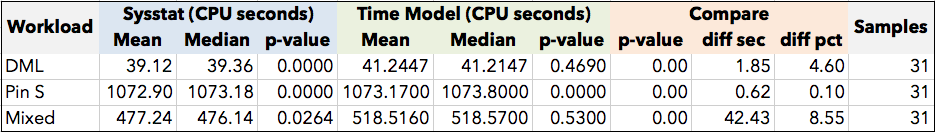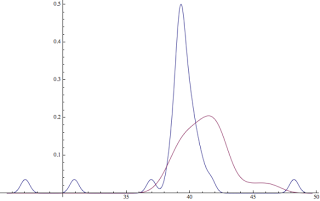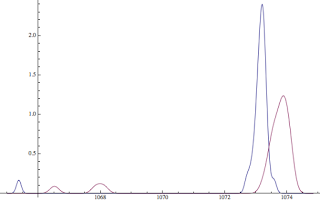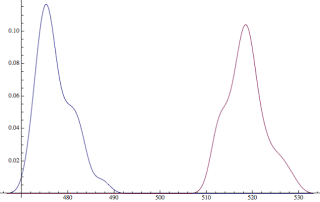## Fixed in Oracle Database 12c? Instance CPU Consumption Reporting via v\$sysstat

Posted on 09-Oct-2013 by Craig Shallahamer, craig@orapub.com

### Is Oracle 12c Instance Level CPU Consumption Reporting Fixed?

I was curious if Oracle had fixed instance level v\$sysstat CPU consumption statistic reporting in 12c. Why was a curious? Because in the past, the Oracle Database 6, 7, 8i, 9i, 10g, 11g have all incorrectly reported instance wide CPU consumption when sourced from the v\$sysstat view. Also, when Stori (OraPub's performance analysis product) pulls CPU consumption, I've got to be confident in the result!

As reported in my Firefighting Book, my tests have shown the time model view (v\$sess_time_model, v\$sys_time_model) CPU consumption for both foreground and background processes are spot on. The lesson was to always use v\$sys_time_model for CPU consumption.

Has this been fixed in Oracle Database 12c? This is what I'm going to find out!

If you don't want to get into the details, here is the results of my experiments:

All three workloads tested showed Oracle Database 12c reported instance wide CPU consumption incorrectly when sourced from the v\$sysstat view statistic, CPU used by this session.

How did I arrive at this conclusion? Read on...

On a related note, last March I posted the results of an experiment about the correctness of SQL statement CPU times. The experiment compared SQL CPU times from four different sources. The results were stunning.

### Experimental Setup

My test is simple. So simple you can copy and paste the below script into your system, as long as it is Oracle Database 10g or higher. You can download the script HERE and it shown below.

``````\$ cat cpu_sys_delta_expr.sql
set tab off
alter system set resource_manager_plan = '';
show parameter resource_manager_plan

drop table op_bogus;
create table op_bogus (sample_no number, ss_sec number, tm_db_cpu_sec number, tm_bg_cpu_sec number);

begin
declare
chill_var   number := 180;
max_sample_var  number := 31;
sample_number_var  number;

t0_ss_sec_var number;
t0_tm_db_cpu_sec_var number;
t0_tm_bg_cpu_sec_var number;
t1_ss_sec_var number;
t1_tm_db_cpu_sec_var number;
t1_tm_bg_cpu_sec_var number;
delta_ss_sec_var number;
delta_tm_db_cpu_sec_var number;
delta_tm_bg_cpu_sec_var number;
begin
delete from op_bogus;

for sample_number_var in 1..max_sample_var
loop

select ss.value/100 ,
stmfg.value/1000000 ,
stmbg.value/1000000
into   t0_ss_sec_var, t0_tm_db_cpu_sec_var, t0_tm_bg_cpu_sec_var
from   v\$sysstat ss,
v\$sys_time_model stmbg,
v\$sys_time_model stmfg
where  stmfg.stat_name = 'DB CPU'
and  stmbg.stat_name = 'background cpu time'
and  ss.name = 'CPU used by this session';

dbms_lock.sleep(chill_var);

select ss.value/100 ,
stmfg.value/1000000 ,
stmbg.value/1000000
into   t1_ss_sec_var, t1_tm_db_cpu_sec_var, t1_tm_bg_cpu_sec_var
from   v\$sysstat ss,
v\$sys_time_model stmbg,
v\$sys_time_model stmfg
where  stmfg.stat_name = 'DB CPU'
and  stmbg.stat_name = 'background cpu time'
and  ss.name = 'CPU used by this session';

delta_ss_sec_var        := t1_ss_sec_var - t0_ss_sec_var ;
delta_tm_db_cpu_sec_var := t1_tm_db_cpu_sec_var - t0_tm_db_cpu_sec_var ;
delta_tm_bg_cpu_sec_var := t1_tm_bg_cpu_sec_var - t0_tm_bg_cpu_sec_var ;

insert into op_bogus values ( sample_number_var, delta_ss_sec_var,
delta_tm_db_cpu_sec_var, delta_tm_bg_cpu_sec_var );

commit;
end loop;
end;
end;
/

select sample_no, ss_sec-(TM_DB_CPU_SEC+TM_BG_CPU_SEC) diff_sec
from   op_bogus
order by 1
/

col np noprint

prompt sysstat results
select sample_no np, ss_sec||',' from op_bogus order by 1;

prompt time model  results
select sample_no np, (TM_DB_CPU_SEC+TM_BG_CPU_SEC)||',' from op_bogus order by 1; ``````

Unlike the time model's v\$sys_time_model view, the v\$sysstat view does not categorize CPU consumption by foreground and by background process. It simply places "all" the CPU consumption into the single statistics, CPU used by this session. Therefore, to compare the CPU consumption values I must sum the v\$sys_time_model DB CPU and background cpu time statistics when comparing to the v\$sysstat CPU used by this session statistic.

Using my free "opload" toolkit (which you can download HERE), I placed three distinct loads (details in next section) on my Oracle Database 12c (12.1.0.1) system. It's a 64 bit six core Linux (2.6.32-300.3.1.el6uek.x86_64) box.

I only recycled the instance before the first workload change. However, between each workload change, I killed all the server/foreground processes and let the system settle down, a verified the settling via the OS command vmstat. For each of the 3 workloads, 31 samples at 180 seconds each were gathered, resulting in 93 samples for a total of 16740 seconds (4.65 hours) of experimental data.

### Experimental Results

To summarize again, Oracle Database 12c v\$sysstat still incorrectly reports Oracle process CPU consumption. Here are the experimental details, which are explained in the sections below.I chose to do the experimental analysis in Mathematica. All the raw experimental results (which are in text files), the Mathematica notepads in both native Mathematica and PDF, the charts, and the data collection script have been zipped into a single file that can be downloaded HERE. There is a LOT more detail in the Mathematic notepads.

Total instance CPU was gathered from three distinct loads were used: DML, Pin S, and Mixed. The DML load consisted of multiple sessions updated and inserting and deleting rows. The Pin S load was the result of multiple sessions opening and closing cursors. There were enough sessions doing this to causes sessions to wait on simply pinning the cursor in shared mode, hence the wait "cursor: pin S". The Mixed workload contains sessions doing queries and some DML. I didn't hammer the system to death, but there was a nice workload mix.

Summary: Oracle Database 12c v\$sysstat and v\$sys_time_model CPU consumption is reported differently.

Details: The average instance CPU consumption for the 31 samples (180 seconds each) from v\$sysstat is 39.12 seconds and the sample set is not normally distributed (p-value = 0.000). The mean for v\$sys_time_model is 41.24 and the sample set is normally distributed (p-value = 0.469).

While that's only a 1.85 second difference, it's also a 4.60% difference. Because both sample set distributions are not normally distributed, a location equivalency test was used to determine if the differences in the means is statistically significant. Mathematica choose to do a Kruskal-Vallis test resulting in a p-value of 0.000 meaning the sample set CPU times from v\$sysstat and v\$sys_time_model are statistically different. Shown together below are their smoothed histograms.This image above shows the two DML sample sets (blue:v\$sysstat, red:v\$sys_time_model) smooth histograms. Statistically the two samples are different and numerically their averages are 4.6% different.

Summary: Oracle Database 12c v\$sysstat and v\$sys_time_model CPU consumption is reported differently.

Details: The average instance CPU consumption for the 31 samples (180 seconds each) from v\$sysstat is 1072.90 seconds and the sample set is not normally distributed (p-value = 0.000). The mean for v\$sys_time_model is 1073.17 and the sample set is not normally distributed (p-value = 0.000).

That is only a 0.62 second difference, which is also a 0.10% difference. Because both sample set distributions are not normally distributed, a location equivalency test was used to determine if the differences in the means is statistically significant. Mathematica choose to do a Kruskal-Vallis test resulting in a p-value of 0.000 meaning the sample set CPU times from v\$sysstat and v\$sys_time_model are statistically different. Shown together below are their smoothed histograms.This image above shows the two Pin S sample sets (blue:v\$sysstat, red:v\$sys_time_model) smooth histograms. Statistically the two samples are different and numerically their averages are 0.10% different.

Summary: Oracle Database 12c v\$sysstat and v\$sys_time_model CPU consumption is reported differently.

Details: The average instance CPU consumption for the 31 samples (180 seconds each) from v\$sysstat is 477.24 seconds and the sample set is not normally distributed (p-value = 0.026). The mean for v\$sys_time_model is 518.52 and the sample set is normally distributed (p-value = 0.530).

That is a shocking 42.43 second difference, which is also a 8.55% difference! Because both sample set distributions are not normally distributed, a location equivalency test was used to determine if the differences in the means is statistically significant. Somewhat interestingly Mathematica chose to do a K-sample Anderson-Darling test resulting in a p-value of 0.000 meaning the sample set CPU times from v\$sysstat and v\$sys_time_model are statistically different. Shown together below are their smoothed histograms.This image above shows the two Mixe sample sets (blue:v\$sysstat, red:v\$sys_time_model) smooth histograms. Statistically the two samples are different and numerically their averages are 8.55% different.

### What Does This Mean?

There are three basic "take aways" from this experiment:

• Oracle Database 12c reports instance level CPU times from v\$sysstat incorrectly.
• Based on my tests from 11g and reported in my Oracle Performance Firefighting book, instance CPU consumption based on v\$sys_time_model is correct.
• When gathering instance wide CPU consumption, use the time model view v\$sys_time_model.